## From ars technica: “Turbulence, the oldest unsolved problem in physics”

10/10/2018
Lee Phillips

The flow of water through a pipe is still in many ways an unsolved problem.

Werner Heisenberg won the 1932 Nobel Prize for helping to found the field of quantum mechanics and developing foundational ideas like the Copenhagen interpretation and the uncertainty principle. The story goes that he once said that, if he were allowed to ask God two questions, they would be, “Why quantum mechanics? And why turbulence?” Supposedly, he was pretty sure God would be able to answer the first question.

The quote may be apocryphal, and there are different versions floating around. Nevertheless, it is true that Heisenberg banged his head against the turbulence problem for several years.

His thesis advisor, Arnold Sommerfeld, assigned the turbulence problem to Heisenberg simply because he thought none of his other students were up to the challenge—and this list of students included future luminaries like Wolfgang Pauli and Hans Bethe. But Heisenberg’s formidable math skills, which allowed him to make bold strides in quantum mechanics, only afforded him a partial and limited success with turbulence.

Some nearly 90 years later, the effort to understand and predict turbulence remains of immense practical importance. Turbulence factors into the design of much of our technology, from airplanes to pipelines, and it factors into predicting important natural phenomena such as the weather. But because our understanding of turbulence over time has stayed largely ad-hoc and limited, the development of technology that interacts significantly with fluid flows has long been forced to be conservative and incremental. If only we became masters of this ubiquitous phenomenon of nature, these technologies might be free to evolve in more imaginative directions.

An undefined definition

Here is the point at which you might expect us to explain turbulence, ostensibly the subject of the article. Unfortunately, physicists still don’t agree on how to define it. It’s not quite as bad as “I know it when I see it,” but it’s not the best defined idea in physics, either.

So for now, we’ll make do with a general notion and try to make it a bit more precise later on. The general idea is that turbulence involves the complex, chaotic motion of a fluid. A “fluid” in physics talk is anything that flows, including liquids, gases, and sometimes even granular materials like sand.

Turbulence is all around us, yet it’s usually invisible. Simply wave your hand in front of your face, and you have created incalculably complex motions in the air, even if you can’t see it. Motions of fluids are usually hidden to the senses except at the interface between fluids that have different optical properties. For example, you can see the swirls and eddies on the surface of a flowing creek but not the patterns of motion beneath the surface. The history of progress in fluid dynamics is closely tied to the history of experimental techniques for visualizing flows. But long before the advent of the modern technologies of flow sensors and high-speed video, there were those who were fascinated by the variety and richness of complex flow patterns.One of the first to visualize these flows was scientist, artist, and engineer Leonardo da Vinci, who combined keen observational skills with unparalleled artistic talent to catalog turbulent flow phenomena. Back in 1509, Leonardo was not merely drawing pictures. He was attempting to capture the essence of nature through systematic observation and description. In this figure, we see one of his studies of wake turbulence, the development of a region of chaotic flow as water streams past an obstacle.

For turbulence to be considered a solved problem in physics, we would need to be able to demonstrate that we can start with the basic equation describing fluid motion and then solve it to predict, in detail, how a fluid will move under any particular set of conditions. That we cannot do this in general is the central reason that many physicists consider turbulence to be an unsolved problem.

I say “many” because some think it should be considered solved, at least in principle. Their argument is that calculating turbulent flows is just an application of Newton’s laws of motion, albeit a very complicated one; we already know Newton’s laws, so everything else is just detail. Naturally, I hold the opposite view: the proof is in the pudding, and this particular pudding has not yet come out right.

The lack of a complete and satisfying theory of turbulence based on classical physics has even led to suggestions that a full account requires some quantum mechanical ingredients: that’s a minority view, but one that can’t be discounted.

An example of why turbulence is said to be an unsolved problem is that we can’t generally predict the speed at which an orderly, non-turbulent (“laminar”) flow will make the transition to a turbulent flow. We can do pretty well in some special cases—this was one of the problems that Heisenberg had some success with—but, in general, our rules of thumb for predicting the transition speeds are summaries of experiments and engineering experience.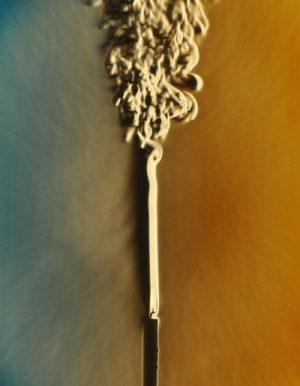There are many phenomena in nature that illustrate the often sudden transformation from a calm, orderly flow to a turbulent flow.The transition to turbulence. Credit: Dr. Gary Settles

This figure above is a nice illustration of this transition phenomenon. It shows the hot air rising from a candle flame, using a 19th century visualization technique that makes gases of different densities look different. Here, the air heated by the candle is less dense than the surrounding atmosphere.

For another turbulent transition phenomenon familiar to anyone who frequents the beach, consider gentle, rolling ocean waves that become complex and foamy as they approach the shore and “break.” In the open ocean, wind-driven waves can also break if the windspeed is high or if multiple waves combine to form a larger one.

For another visual aid, there is a centuries-old tradition in Japanese painting of depicting turbulent, breaking ocean waves. In these paintings, the waves are not merely part of the landscape but the main subjects. These artists seemed to be mainly concerned with conveying the beauty and terrible power of the phenomenon, rather than, as was Leonardo, being engaged in a systematic study of nature. One of the most famous Japanese artworks, and an iconic example of this genre, is Hokusai’s “Great Wave,” a woodblock print published in 1831.Hokusai’s “Great Wave.”

For one last reason to consider turbulence an unsolved problem, turbulent flows exhibit a wide range of interesting behavior in time and space. Most of these have been discovered by measurement, not predicted, and there’s still no satisfying theoretical explanation for them.

Simulation

Reasons for and against “mission complete” aside, why is the turbulence problem so hard? The best answer comes from looking at both the history and current research directed at what Richard Feynman once called “the most important unsolved problem of classical physics.”

The most commonly used formula for describing fluid flow is the Navier-Stokes equation. This is the equation you get if you apply Newton’s first law of motion, F = ma (force = mass × acceleration), to a fluid with simple material properties, excluding elasticity, memory effects, and other complications. Complications like these arise when we try to accurately model the flows of paint, polymers, some biological fluids such as blood (there are many other substances also that violate the assumptions of the Navier-Stokes equations). But for water, air, and other simple liquids and gases, it’s an excellent approximation.

The Navier-Stokes equation is difficult to solve because it is nonlinear. This word is thrown around quite a bit, but here it means something specific. You can build up a complicated solution to a linear equation by adding up many simple solutions. An example you may be aware of is sound: the equation for sound waves is linear, so you can build up a complex sound by adding together many simple sounds of different frequencies (“harmonics”). Elementary quantum mechanics is also linear; the Schrödinger equation allows you to add together solutions to find a new solution.

But fluid dynamics doesn’t work this way: the nonlinearity of the Navier-Stokes equation means that you can’t build solutions by adding together simpler solutions. This is part of the reason that Heisenberg’s mathematical genius, which served him so well in helping to invent quantum mechanics, was put to such a severe test when it came to turbulence.

Heisenberg was forced to make various approximations and assumptions to make any progress with his thesis problem. Some of these were hard to justify; for example, the applied mathematician Fritz Noether (a brother of Emmy Noether) raised prominent objections to Heisenberg’s turbulence calculations for decades before finally admitting that they seemed to be correct after all.

(The situation was so hard to resolve that Heisenberg himself said, while he thought his methods were justified, he couldn’t find the flaw in Fritz Noether’s reasoning, either!)

The cousins of the Navier-Stokes equation that are used to describe more complex fluids are also nonlinear, as is a simplified form, the Euler equation, that omits the effects of friction. There are cases where a linear approximation does work well, such as flow at extremely slow speeds (imagine honey flowing out of a jar), but this excludes most problems of interest including turbulence.

Who’s down with CFD?

Despite the near impossibility of finding mathematical solutions to the equations for fluid flows under realistic conditions, science still needs to get some kind of predictive handle on turbulence. For this, scientists and engineers have turned to the only option available when pencil and paper failed them—the computer. These groups are trying to make the most of modern hardware to put a dent in one of the most demanding applications for numerical computing: calculating turbulent flows.

The need to calculate these chaotic flows has benefited from (and been a driver of) improvements in numerical methods and computer hardware almost since the first giant computers appeared. The field is called computational fluid dynamics, often abbreviated as CFD.

Early in the history of CFD, engineers and scientists applied straightforward numerical techniques in order to try to directly approximate solutions to the Navier-Stokes equations. This involves dividing up space into a grid and calculating the fluid variables (pressure, velocity) at each grid point. The problem of the large range of spatial scales immediately makes this approach expensive: you need to find a solution where the flow features are accurate for the largest scales—meters for pipes, thousands of kilometers for weather, and down to near the molecular scale. Even if you cut off the length scale at the small end at millimeters or centimeters, you will still need millions of grid points.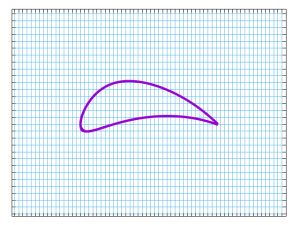A possible grid for calculating the flow over an airfoil.

One approach to getting reasonable accuracy with a manageable-sized grid begins with the realization that there are often large regions where not much is happening. Put another way, in regions far away from solid objects or other disturbances, the flow is likely to vary slowly in both space and time. All the action is elsewhere; the turbulent areas are usually found near objects or interfaces.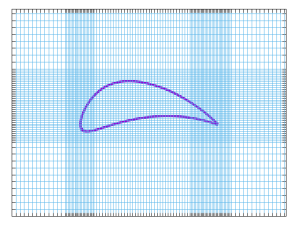A non-uniform grid for calculating the flow over an airfoil.

If we take another look at our airfoil and imagine a uniform flow beginning at the left and passing over it, it can be more efficient to concentrate the grid points near the object, especially at the leading and trailing edges, and not “waste” grid points far away from the airfoil. The next figure shows one possible gridding for simulating this problem.

This is the simplest type of 2D non-uniform grid, containing nothing but straight lines. The state of the art in nonuniform grids is called adaptive mesh refinement (AMR), where the mesh, or grid, actually changes and adapts to the flow during the simulation. This concentrates grid points where they are needed, not wasting them in areas of nearly uniform flow. Research in this field is aimed at optimizing the grid generation process while minimizing the artificial effects of the grid on the solution. Here it’s used in a NASA simulation of the flow around an oscillating rotor blade. The color represents vorticity, a quantity related to angular momentum.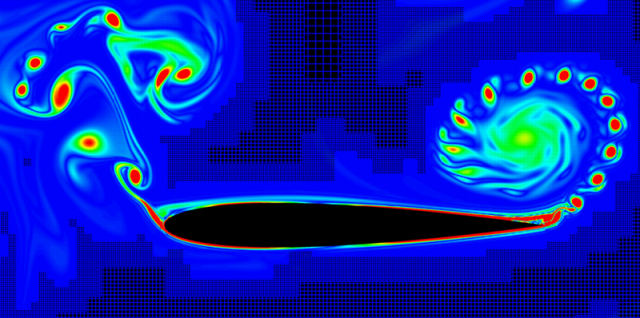Using AMR to simulate the flow around a rotor blade.Neal M. Chaderjian, NASA/Ames

The above image shows the computational grid, rendered as blue lines, as well as the airfoil and the flow solution, showing how the grid adapts itself to the flow. (The grid points are so close together at the areas of highest grid resolution that they appear as solid blue regions.) Despite the efficiencies gained by the use of adaptive grids, simulations such as this are still computationally intensive; a typical calculation of this type occupies 2,000 compute cores for about a week.

Dimitri Mavriplis and his collaborators at the Mavriplis CFD Lab at the University of Wyoming have made available several videos of their AMR simulations.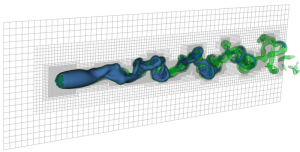AMR simulation of flow past a sphere.Mavriplis CFD Lab

Above is a frame from a video of a simulation of the flow past an object; the video is useful for getting an idea of how the AMR technique works, because it shows how the computational grid tracks the flow features.

This work is an example of how state-of-the-art numerical techniques are capable of capturing some of the physics of the transition to turbulence, illustrated in the image of candle-heated air above.

Another approach to getting the most out of finite computer resources involves making alterations to the equation of motion, rather than, or in addition to, altering the computational grid.

Since the first direct numerical simulations of the Navier-Stokes equations were begun at Los Alamos in the late 1950s, the problem of the vast range of spatial scales has been attacked by some form of modeling of the flow at small scales. In other words, the actual Navier-Stokes equations are solved for motion on the medium and large scales, but, below some cutoff, a statistical or other model is substituted.

The idea is that the interesting dynamics occur at larger scales, and grid points are placed to cover these. But the “subgrid” motions that happen between the gridpoints mainly just dissipate energy, or turn motion into heat, so don’t need to be tracked in detail. This approach is also called large-eddy simulation (LES), the term “eddy” standing in for a flow feature at a particular length scale.

The development of subgrid modeling, although it began with the beginning of CFD, is an active area of research to this day. This is because we always want to get the most bang for the computer buck. No matter how powerful the computer, a sophisticated numerical technique that allows us to limit the required grid resolution will enable us to handle more complex problems.

There are several other prominent approaches to modeling fluid flows on computers, some of which do not make use of grids at all. Perhaps the most successful of these is the technique called “smoothed particle hydrodynamics,” which, as its name suggests, models the fluid as a collection of computational “particles,” which are moved around without the use of a grid. The “smoothed” in the name comes from the smooth interpolations between particles that are used to derive the fluid properties at different points in space.

Theory and experiment

Despite the impressive (and ever-improving) ability of fluid dynamicists to calculate complex flows with computers, the search for a better theoretical understanding of turbulence continues, for computers can only calculate flow solutions in particular situations, one case at a time. Only through the use of mathematics do physicists feel that they’ve achieved a general understanding of a group of related phenomena. Luckily, there are a few main theoretical approaches to turbulence, each with some interesting phenomena they seek to penetrate.

Only a few exact solutions of the Navier-Stokes equations are known; these describe simple, laminar flows (and certainly not turbulent flows of any kind). For flow in a pipe or between two flat plates, the flow velocity profile between two plates is zero at the boundaries and a maximum half-way between them. This parabolic flow profile (shown below) solves the equations: something that has been known for over a century. Laminar flow in a pipe is similar, with the maximum velocity occurring at the center.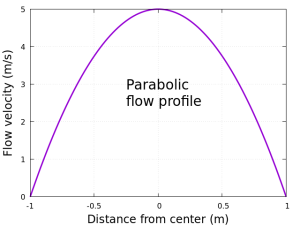Exact solution for flow between plates.

The interesting thing about this parabolic solution, and similar exact solutions, is that they are valid (mathematically speaking) at any flow velocity, no matter how high. However, experience shows that while this works at low speeds, the flow breaks up and becomes turbulent at some moderate “critical” speed. Using mathematical methods to try to find this critical speed is part of what Heisenberg was up to in his thesis work.

Theorists describe what’s happening here by using the language of stability theory. Stability theory is the examination of the exact solutions to the Navier-Stokes equation and their ability to survive “perturbations,” which are small disturbances added to the flow. These disturbances can be in the form of boundaries that are less than perfectly smooth, variations in the pressure driving the flow, etc.

The idea is that, while the low-speed solution is valid at any speed, near a critical speed another solution also becomes valid, and nature prefers that second, more complex solution. In other words, the simple solution has become unstable and is replaced by a second one. As the speed is ramped up further, each solution gives way to a more complicated one, until we arrive at the chaotic flow we call turbulence.

In the real world, this will always happen, because perturbations are always present—and this is why laminar flows are much less common in everyday experience than turbulence.

Experiments to directly observe these instabilities are delicate, because the distance between the first instability and the onset of full-blown turbulence is usually quite small. You can see a version of the process in the figure above, showing the transition to turbulence in the heated air column above a candle. The straight column is unstable, but it takes a while before the sinuous instability grows large enough for us to see it as a visible wiggle. Almost as soon as this happens, the cascade of instabilities piles up, and we see a sudden explosion into turbulence.

Another example of the common pattern is in the next illustration, which shows the typical transition to turbulence in a flow bounded by a single wall.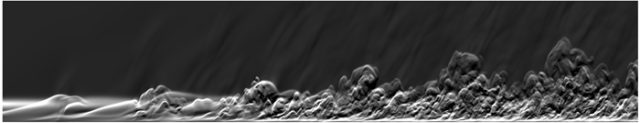Transition to turbulence in a wall-bounded flow. NASA.

We can again see an approximately periodic disturbance to the laminar flow begin to grow, and after just a few wavelengths the flow suddenly becomes turbulent.

Capturing, and predicting, the transition to turbulence is an ongoing challenge for simulations and theory; on the theoretical side, the effort begins with stability theory.

In fluid flows close to a wall, the transition to turbulence can take a somewhat different form. As in the other examples illustrated here, small disturbances get amplified by the flow until they break down into chaotic, turbulent motion. But the turbulence does not involve the entire fluid, instead confining itself to isolated spots, which are surrounded by calm, laminar flow. Eventually, more spots develop, enlarge, and ultimately merge, until the entire flow is turbulent.

The fascinating thing about these spots is that, somehow, the fluid can enter them, undergo a complex, chaotic motion, and emerge calmly as a non-turbulent, organized flow on the other side. Meanwhile, the spots persist as if they were objects embedded in the flow and attached to the boundary.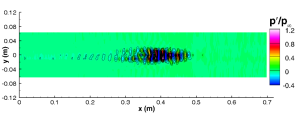Turbulent spot experiment: pressure fluctuation. (Credit: Katya Casper et al., Sandia National Labs)

Despite a succession of first-rate mathematical minds puzzling over the Navier-Stokes equation since it was written down almost two centuries ago, exact solutions still are rare and cherished possessions, and basic questions about the equation remain unanswered. For example, we still don’t know whether the equation has solutions in all situations. We’re also not sure if its solutions, which supposedly represent the real flows of water and air, remain well-behaved and finite, or whether some of them blow up with infinite energies or become unphysically unsmooth.

The scientist who can settle this, either way, has a cool million dollars waiting for them—this is one of the seven unsolved “Millennium Prize” mathematical problems set by the Clay Mathematics Institute.

Fortunately, there are other ways to approach the theory of turbulence, some of which don’t depend on the knowledge of exact solutions to the equations of motion. The study of the statistics of turbulence uses the Navier-Stokes equation to deduce average properties of turbulent flows without trying to solve the equations exactly. It addresses questions like, “if the velocity of the flow here is so and so, then what is the probability that the velocity one centimeter away will be within a certain range?” It also answers questions about the average of quantities such as the resistance encountered when trying to push water through a pipe, or the lifting force on an airplane wing.

These are the quantities of real interest to the engineer, who has little use for the physicist’s or mathematician’s holy grail of a detailed, exact description.

Despite a succession of first-rate mathematical minds puzzling over the Navier-Stokes equation since it was written down almost two centuries ago, exact solutions still are rare and cherished possessions, and basic questions about the equation remain unanswered. For example, we still don’t know whether the equation has solutions in all situations. We’re also not sure if its solutions, which supposedly represent the real flows of water and air, remain well-behaved and finite, or whether some of them blow up with infinite energies or become unphysically unsmooth.

The scientist who can settle this, either way, has a cool million dollars waiting for them—this is one of the seven unsolved “Millennium Prize” mathematical problems set by the Clay Mathematics Institute.

Fortunately, there are other ways to approach the theory of turbulence, some of which don’t depend on the knowledge of exact solutions to the equations of motion. The study of the statistics of turbulence uses the Navier-Stokes equation to deduce average properties of turbulent flows without trying to solve the equations exactly. It addresses questions like, “if the velocity of the flow here is so and so, then what is the probability that the velocity one centimeter away will be within a certain range?” It also answers questions about the average of quantities such as the resistance encountered when trying to push water through a pipe, or the lifting force on an airplane wing.

These are the quantities of real interest to the engineer, who has little use for the physicist’s or mathematician’s holy grail of a detailed, exact description.

It turns out that the one great obstacle in the way of a statistical approach to turbulence theory is, once again, the nonlinear term in the Navier-Stokes equation. When you use this equation to derive another equation for the average velocity at a single point, it contains a term involving something new: the velocity correlation between two points. When you derive the equation for this velocity correlation, you get an equation with yet another new term: the velocity correlation involving three points. This process never ends, as the diabolical nonlinear term keeps generating higher-order correlations.

The need to somehow terminate, or “close,” this infinite sequence of equations is known as the “closure problem” in turbulence theory and is still the subject of active research. Very briefly, to close the equations you need to step outside of the mathematical procedure and appeal to a physically motivated assumption or approximation.

Despite its difficulty, some type of statistical solution to the fluid equations is essential for describing the phenomena of fully developed turbulence, of which there are a number. Turbulence need not be merely a random, featureless expanse of roiling fluid; in fact, it usually is more interesting than that. One of the most intriguing phenomena is the existence of persistent, organized structures within a violent, chaotic flow environment. We are all familiar with magnificent examples of these in the form of the storms on Jupiter, recognizable, even iconic, features that last for years, embedded within a highly turbulent flow.

More down-to-Earth examples occur in almost any real-world case of a turbulent flow—in fact, experimenters have to take great pains if they want to create a turbulent flow field that is truly homogeneous, without any embedded structure.

In the below images of a turbulent wake behind a cylinder and of the transition to turbulence in a wall-bounded flow, you can see the echoes of the wave-like disturbance that precedes the onset of fully developed turbulence: a periodicity that persists even as the flow becomes chaotic.Cyclones at Jupiter’s north pole. NASA, JPL-Caltech, SwRI, ASI, INAF, JIRAM.Wake behind a cylinder. Joseph Straccia et al. (CC By NC-ND)

When your basic governing equation is very hard to solve or even to simulate, it’s natural to look for a more tractable equation or model that still captures most of the important physics. Much of the theoretical effort to understand turbulence is of this nature.

We’ve mentioned subgrid models above, used to reduce the number of grid points required in a numerical simulation. Another approach to simplifying the Navier-Stokes equation is a class of models called “shell models.” Roughly speaking, in these models you take the Fourier transform of the Navier-Stokes equation, leading to a description of the fluid as a large number of interacting waves at different wavelengths. Then, in a systematic way, you discard most of the waves, keeping just a handful of significant ones. You can then calculate, using a computer or, with the simplest models, by hand, the mode interactions and the resulting turbulent properties. While, naturally, much of the physics is lost in these types of models, they allow some aspects of the statistical properties of turbulence to be studied in situations where the full equations cannot be solved.

Occasionally, we hear about the “end of physics”—the idea that we are approaching the stage where all the important questions will be answered, and we will have a theory of everything. But from another point of view, the fact that such a commonplace phenomenon as the flow of water through a pipe is still in many ways an unsolved problem means that we are unlikely to ever reach a point that all physicists will agree is the end of their discipline. There remains enough mystery in the everyday world around us to keep physicists busy far into the future.five-ways-keep-your-child-safe-school-shootings